Introduction:

Analysis of “Breast Cancer Wisconsin (Diagnostic) Data Set”. The dataset is available from “UCI Machine Learning Repository”. Data used is “breast-cancer-wisconsin.data”" (1) and “breast-cancer-wisconsin.names”(2).

The dataset has 11 variables with 699 observations, first variable is the identifier and has been excluded in the analyis. Thus, there are 9 predictors and a response variable (class). The response variable denotes “Malignant” or “Benign” cases.

Predictor variables:

• Clump Thickness
• Uniformity of Cell Size
• Single Epithelial Cell Size
• Bare Nuclei
• Uniformity of Cell Shape
• Bland Chromatin
• Mitoses
• Normal Nucleoli

Response variable:

Class - (2 for benign, 4 for malignant)

There are 16 observations where data is incomplete. In further analysis, these cases are imputed (substitued by most likely values) or ignored. In total, there are 241 cases of malignancy, where as benign cases are 458.

Detailed summary of all predictors and response variables are as following.

##  clump_thickness  uniformity_cell_size uniformity_cell_shape
##  Min.   : 1.000   Min.   : 1.000       Min.   : 1.000
##  1st Qu.: 2.000   1st Qu.: 1.000       1st Qu.: 1.000
##  Median : 4.000   Median : 1.000       Median : 1.000
##  Mean   : 4.418   Mean   : 3.134       Mean   : 3.207
##  3rd Qu.: 6.000   3rd Qu.: 5.000       3rd Qu.: 5.000
##  Max.   :10.000   Max.   :10.000       Max.   :10.000
##
##  marginal_adhesion single_epith_cell_size bland_chromatim
##  Min.   : 1.000    Min.   : 1.000         Min.   : 1.000
##  1st Qu.: 1.000    1st Qu.: 2.000         1st Qu.: 2.000
##  Median : 1.000    Median : 2.000         Median : 3.000
##  Mean   : 2.807    Mean   : 3.216         Mean   : 3.438
##  3rd Qu.: 4.000    3rd Qu.: 4.000         3rd Qu.: 5.000
##  Max.   :10.000    Max.   :10.000         Max.   :10.000
##
##  normal_nucleoli     mitoses        Bare_Nuclei           Class
##  Min.   : 1.000   Min.   : 1.000   Min.   : 1.000   Benign   :458
##  1st Qu.: 1.000   1st Qu.: 1.000   1st Qu.: 1.000   Malignant:241
##  Median : 1.000   Median : 1.000   Median : 1.000
##  Mean   : 2.867   Mean   : 1.589   Mean   : 3.545
##  3rd Qu.: 4.000   3rd Qu.: 1.000   3rd Qu.: 6.000
##  Max.   :10.000   Max.   :10.000   Max.   :10.000
##                                    NA's   :16

Exploratory Analysis

Histograms

Following is the pictorial representation of occurrences of Malignant/Benign cases based on the variables. Figures are categorized in such a way that we can understand what is distribution of various variables. E.g. it appears that Malign cases decrease and Benign cases increase as clump_thickness increases.For Bare Nuclei, this can be seen that malignant cases increase with increase in this variable. The higher the bands are, more the number of occurrences for that type.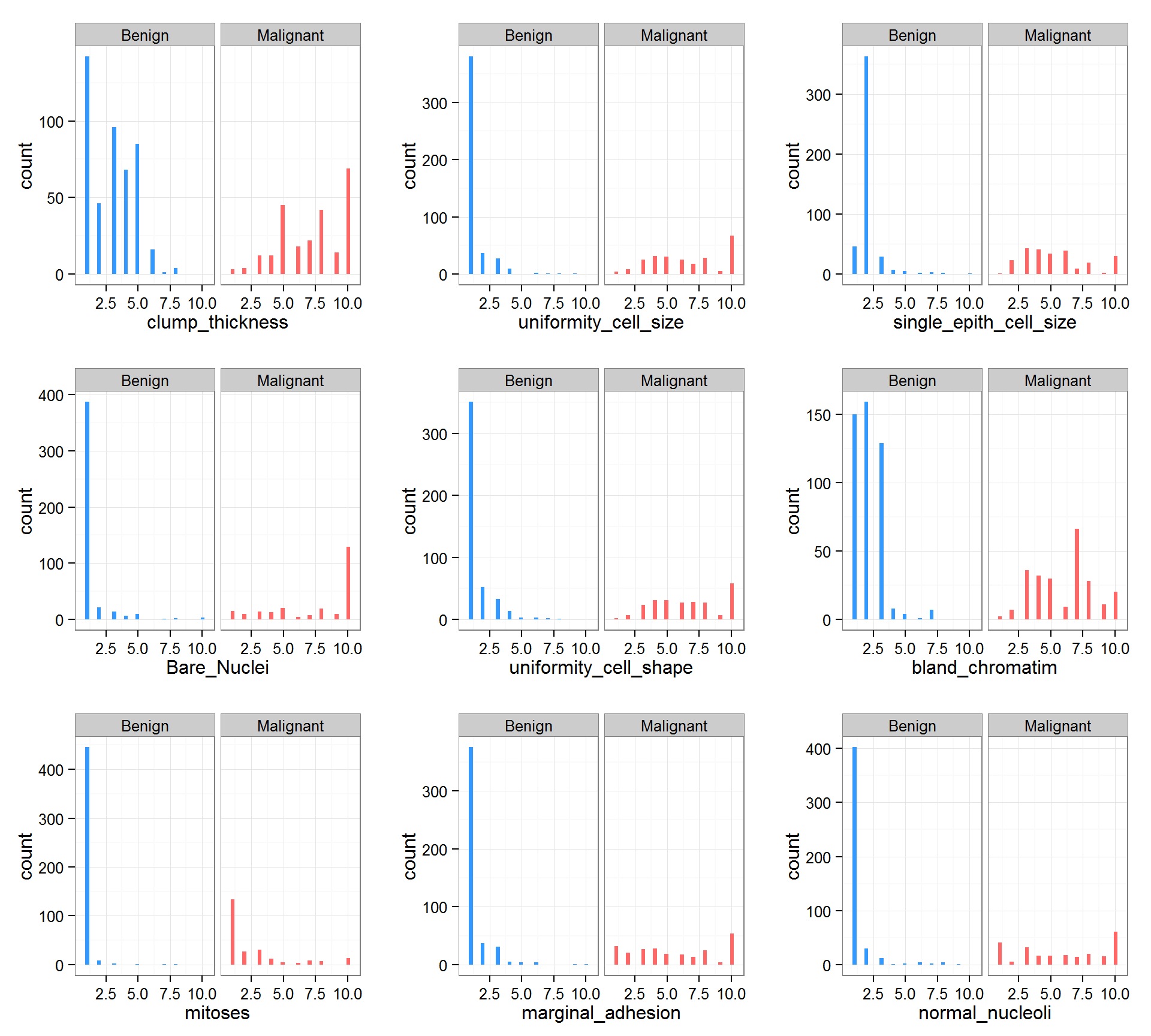Boxplots

Boxplots(3) are another way of showing the various attributes. With the help of these, we can understand what are the median values for Benign or Malignant cases. e.g, in the case of “Bare Nuclei”, the median value is 1.0 for Benign cases, and 10.0 for Malignant ones. The dots tell that there are some values which are outliers, exception cases. An observation here is that, the median value of various variables is much higher in Malignant cases.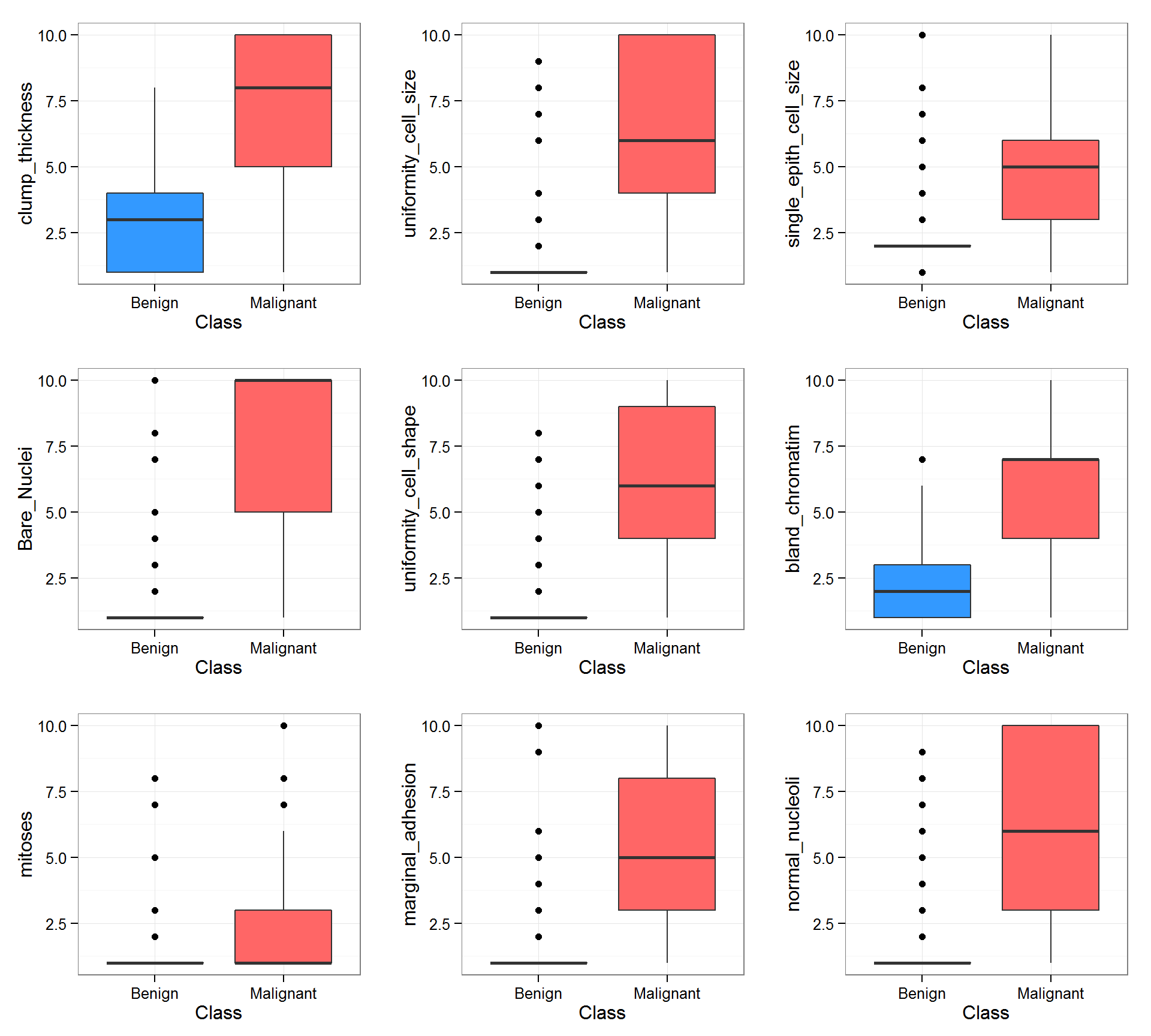Correlations

Correlation helps to understand if there are relationships between variables. If there is a high correlation, then one of the variables would have less impact on the analysis. Following figure provides the relationship between the variables of Wisconsin Data. A circle provides the intersection of two variables. High shaded area denotes high correlation. e.g, marginal_adhesion and uniformity_cell_size show strong relationship.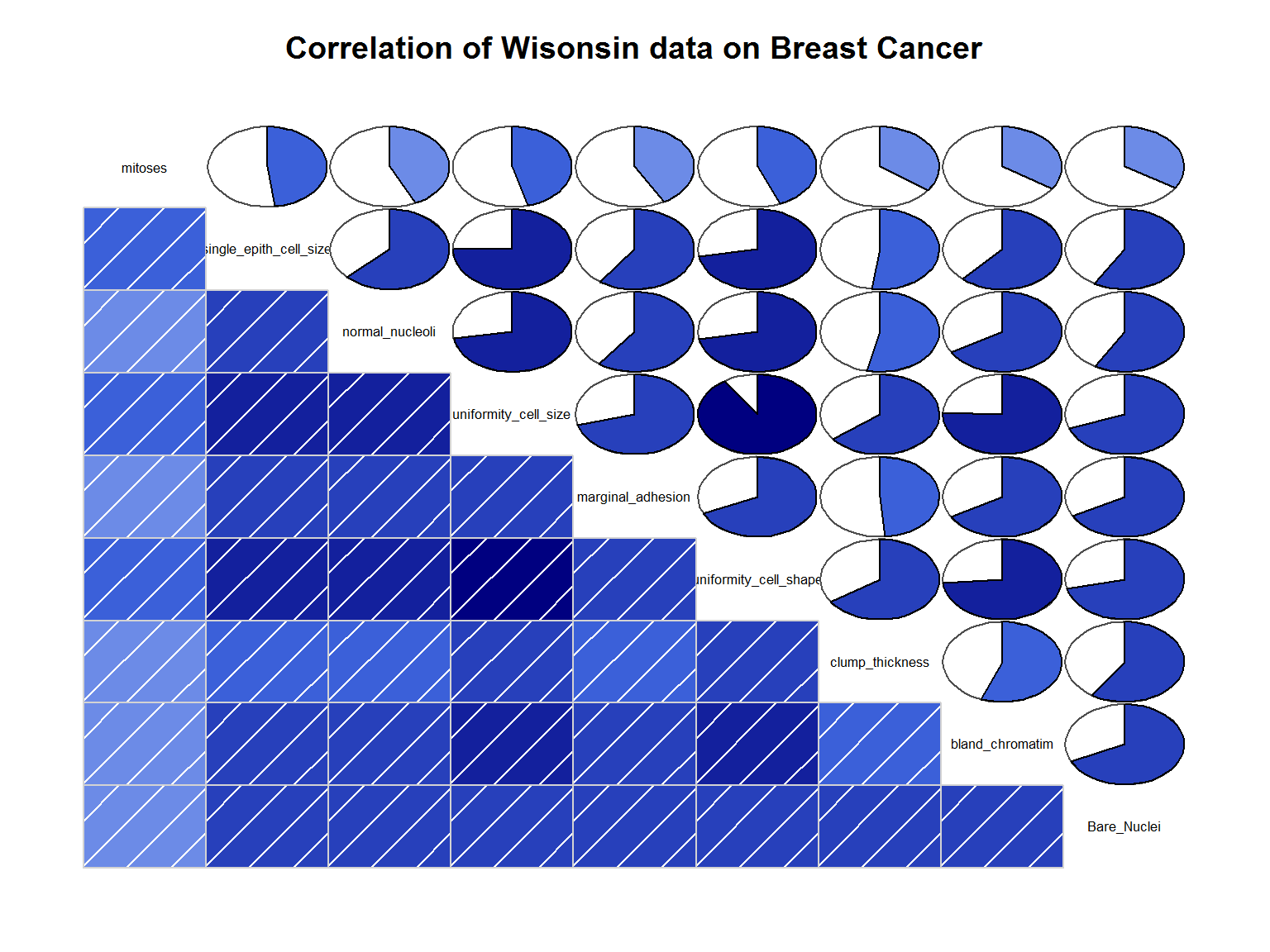Singular Value Decomposition

Singular Value Decomposition or SVD (4) is a technique to reduce the number of variables without losing the features of a dataset. This is especially useful when there is large amount of data to process, as it saves time and computing power. Though our dataset is a small one, we are doing this analysis to identify any pattern.

After doing SVD, it is found out whether the given dataset can be reduced to lesser number of variables. Following plot confirms that with couple of representative variables, the dataset converges into two distinct clusters, Malignant and Benign, with few overlapping values.

Note: The benign area appears to have lesser number of cases, this is due to overlapping of points. The adjoining bar chart provides the actual distribution of this dataset.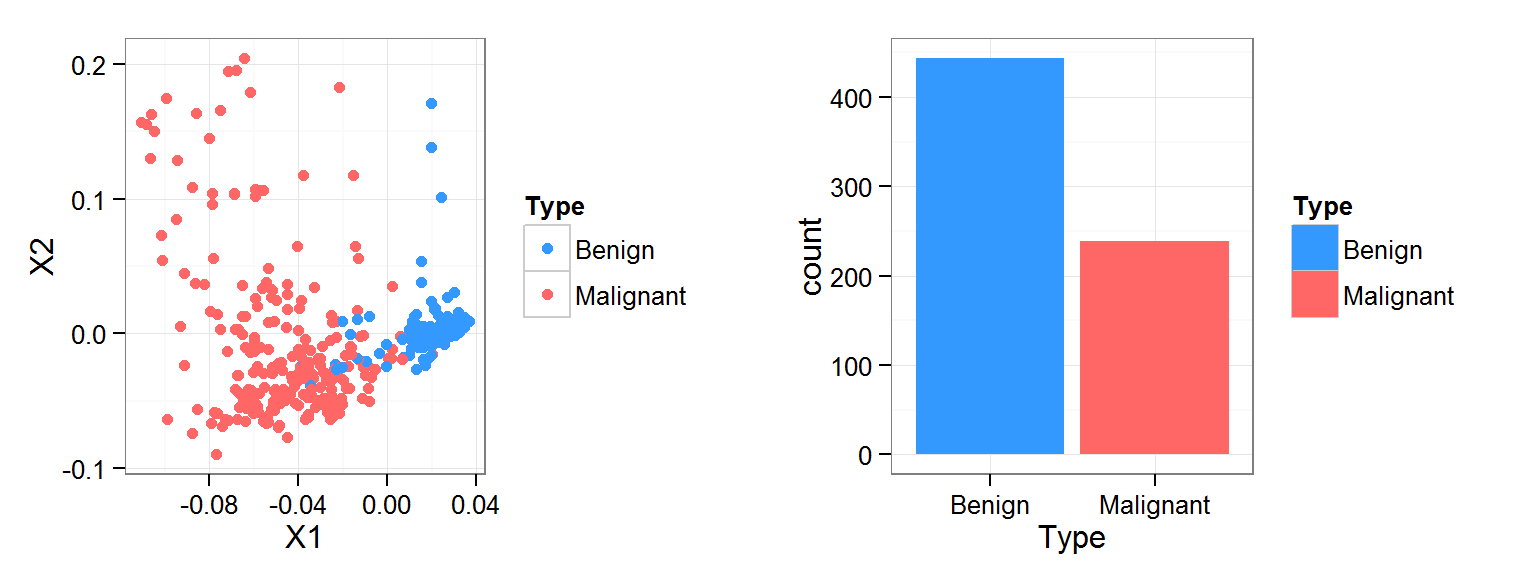Clustering wih Dendrograms

After SVD, clustering techniques are used to evaluate variable performance. With the help of clustering techniques, it is determined whether the data points converge into distinct clusters. Couple of plots are drawn, one with lesser number of variables and the second, which includes all variables. As the plot provides two distinct clusters, with some overlap, the second plot looks more complete. Hence, all variables should be considered for analysis.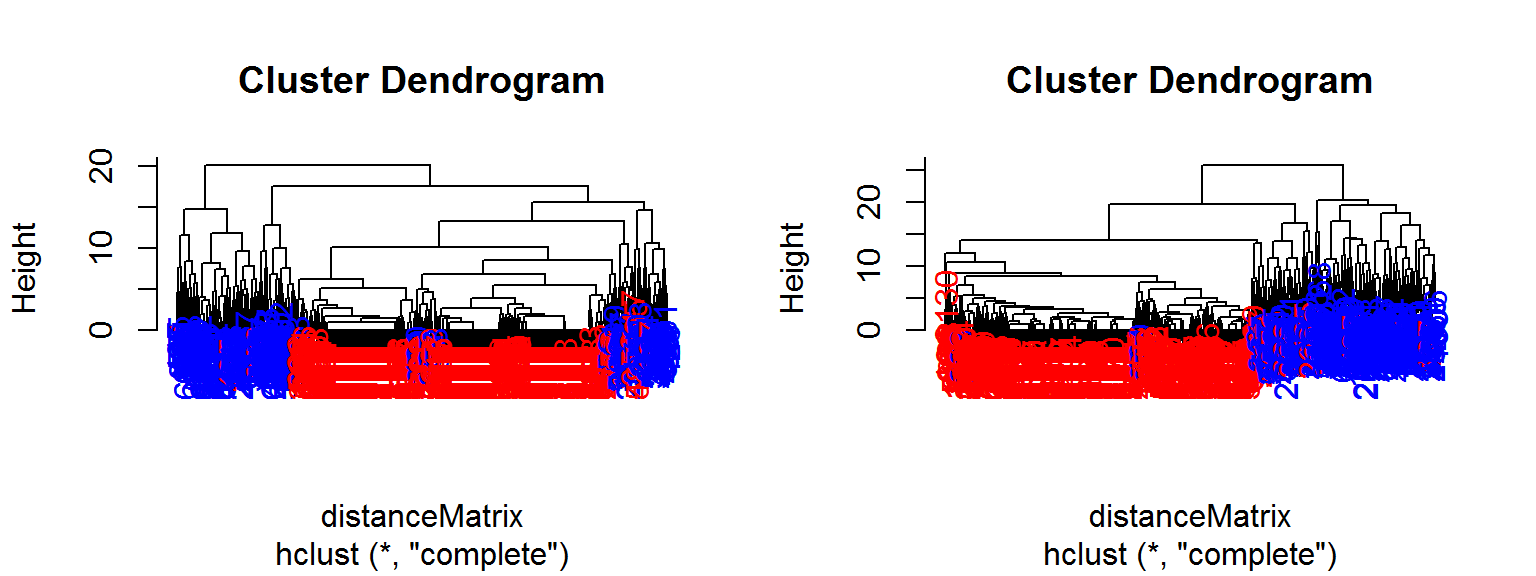Machine Learning Algortihms

K Means Clustering for classification of data

K Means Clustering(5) is an algorithm, which is used to create clusters within a data set. Though these are primarily used in unsupervised(unlabeled) learning, we are using it here to see whether it is possible to map various clusters to a Benign or Malignant category. The algorithm has been run for a number of 50 clusters and error rate was recorded. It was found that a model with 37 clusters provides a lower error rate. The number of clusters required for a binary classification (Maignant/Benign) is too high to do further analysis.

Following is a pictorial representation of error over cluster size.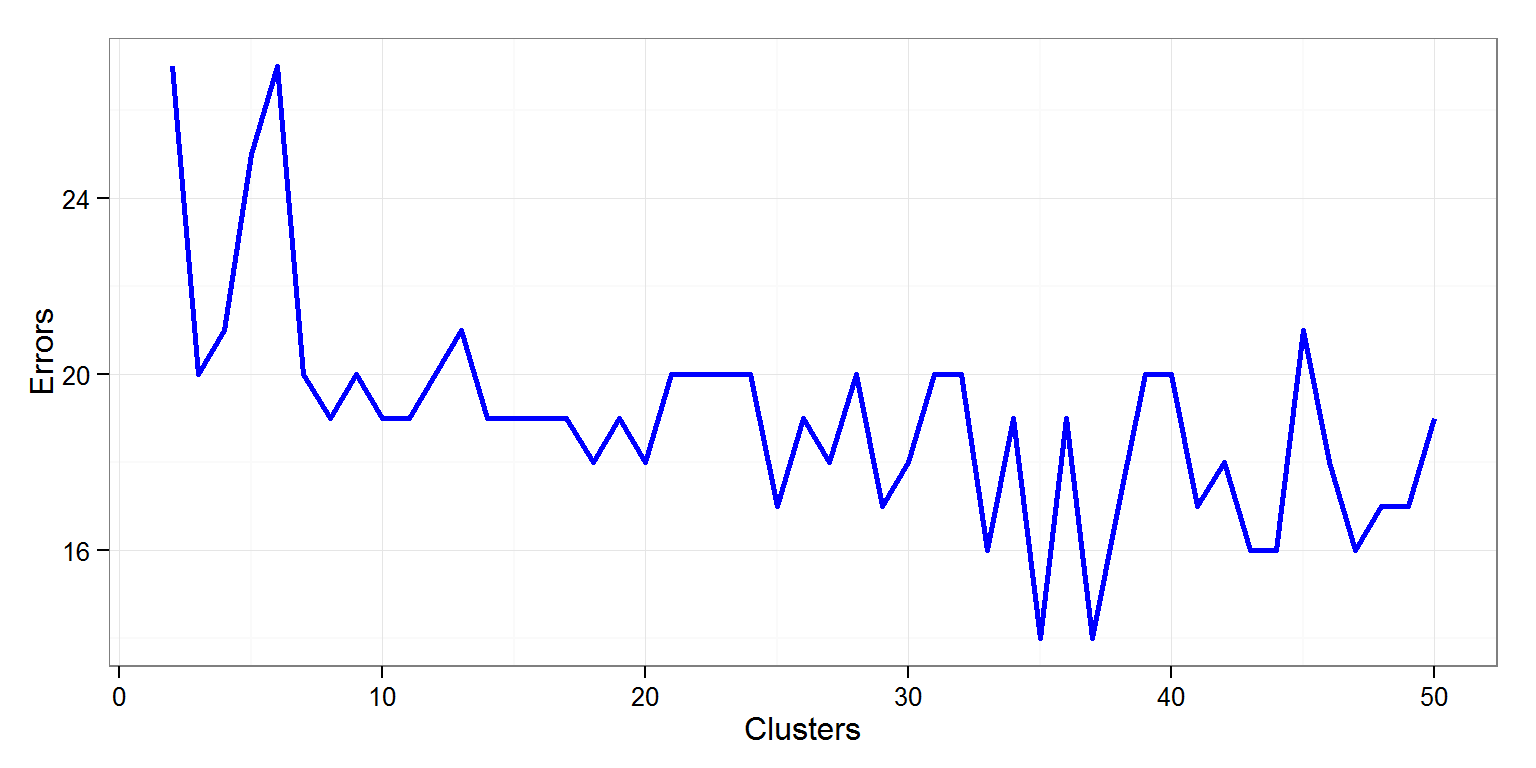Random Forests

Couple of models were built using Random Forests(6). One with including all variables and another with leaving out those variables which were not important. This was found out after analyzing variables from full model.

Building a Random Forest classifier (full):

A Random Forest classifier has been built with all the variables. 20% of the data was kept aside for validation and checking out of sample errors and model was built with 80% of data. This model was then tested on the 20% of the data. The error rate was found to be 5.04.

Building a Random Forest classifier (partial):

This classifier has been built by leaving clump_thickness, normal_nucleoli, mitoses and marginal_adhesion out. This was built on 80% of data. This model was then tested on the 20% of the data. The error rate was found to be 8.63. As the error percentage has increased on out of sample errors, the full model should be considered for final analysis. Variable importance was decided as suggested by Max Kuhn(9).

Decision Trees

Similar to Random Forests, Couple of models were built using Decision Trees(7). One with including all variables and another with leaving out those variables which were not important. These variables were found after analyzing variables from full model.

Building a Rpart (Decision Tree) classifier (full):

A Decision tree classifier has been built with all the variables. 20% of the data was kept aside for validation and checking out of sample errors, and the model was built on 80% of data. The error rate was found to be 9.35.

Building a Rpart (Decision Tree) classifier (partial):

A Decision tree classifier has been built leaving out the variables marginal_adhesion, clump_thickness, mitoses and normal_nucleoli . 20% of the data was kept aside for validation and checking out of sample errors. Model was built on 80% of data. The error rate was found to be 10.07.

K Nearest Neighbours (KNN)

Similar to above, Couple of models were built using KNN(7). One with including all variables and another with leaving out those variables which were not important. These variables were found after analyzing variables from full model.

Building a KNN classifier (with all variables):

A KNN classifier has been built with all the variables. 20% of the data was kept aside for validation and checking out of sample errors. The model was built on 80% of data. The error rate was found to be 6.47.

Building a KNN classifier (Partial):

Based on the variable importane obtained from the full classfier, A KNN classifier with lesser number of variables has been built.For this classifier, mitoses, marginal_adhesion and normal_nuleoli have been removed. 20% of the data was kept aside for validation and checking out of sample errors. The model was built with 80% of data. The error rate was found to be 2.88.

Inference

Three different algorithms for classification are tried - with all varibles and with variable selection. Generally, it is found that errors were increased when variables were decreased, with an exception. With KNN, a model with lesser number of variables has performed better than all other models. As this is a small dataset, this could be a case of overfitting. Second option is to use Random Forest full classifier, as it does better than the KNN Classifier with all variables. Decision trees are showing high error rate, so this could be ignored.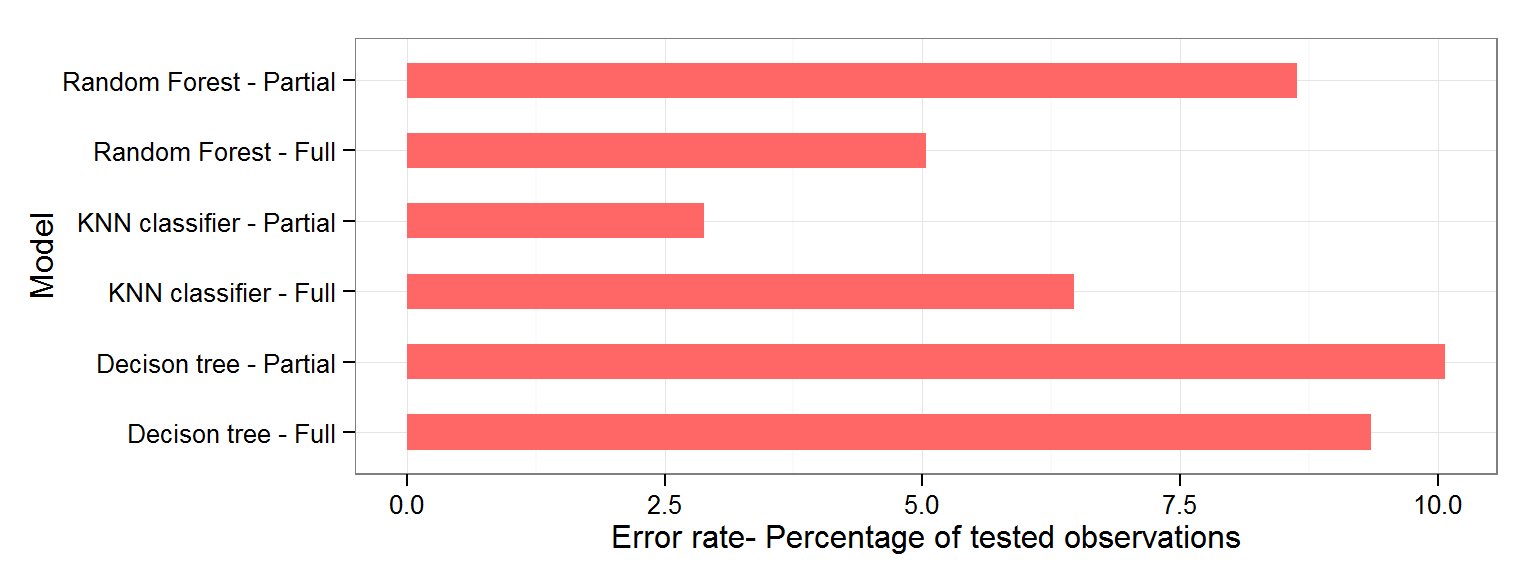Toolkit Used:

Following are the main R Packages and Toolkits have been used to carry out the analysis.

1. Caret - Classification and Regressin Trees.
2. ggplot2. - Majority of figures are created using this package.
3. randomForest.